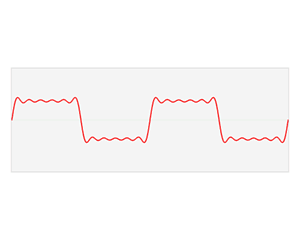# 图卷积,图卷积神经网络$(f*g)(t)={\int}_{-\infty}^{\infty}f(\tau)g(t-\tau) (连续形式)$$(f*g)(t)={\sum}_{\tau=-\infty}^{\infty}f(\tau)g(t-\tau) (离散形式)$实例:掷骰子问题

$$f$$ 向上为1，$$g$$ 向上为3； $$f$$ 向上为2，$$g$$ 向上为2； $$f$$ 向上为3，$$g$$ 向上为1；$\mathbf{h}_{v}^{l+1}=U_{l+1}(\mathbf{h}_v,\sum_{u{\in}ne[v]}M_{l+1}(\mathbf{h}_v^l,\mathbf{h}_u^l,\mathbf{x}_{vu}))$MPNN很好地概括了空域卷积的过程，但定义在这个框架下的所有模型都有一个共同的缺陷：卷积操作针对的对象是整张图，也就意味着要将所有结点放入内存/显存中，才能进行卷积操作。但对实际场景中的大规模图而言，整个图上的卷积操作并不现实。GraphSage提出的动机之一就是解决这个问题。从该方法的名字我们也能看出，区别于传统的全图卷积，GraphSage利用采样(Sample)部分结点的方式进行学习。当然，即使不需要整张图同时卷积，GraphSage仍然需要聚合邻居结点的信息，即论文中定义的aggregate的操作。这种操作类似于MPNN中的消息传递过程。$\mathbf{h}_{v}^{l+1}=\sigma(\mathbf{W}^{l+1}\cdot aggregate(\mathbf{h}_v^l,\{\mathbf{h}_u^l\}),{\forall}u{\in}ne[v])$

GraphSage的设计重点就放在了$$aggregate$$函数的设计上。它可以是不带参数的$$max$$, $$mean$$, 也可以是带参数的如$$LSTM$$等神经网络。核心的原则仍然是，它需要可以处理变长的数据。在本系列博客的第三篇笔者会介绍卷积神经网络中针对图任务的ReadOut操作，$$aggregate$$函数的设计与其有异曲同工之妙，此处就不展开叙述了。

PATCHY-SAN 通过以下三个步骤来解决这两个问题：$(f*g)(t)=F^{-1}[F[f(t)]{\odot}F[g(t)]]$$\hat{f}(t)={\int}f(x){\exp}^{-2{\pi}ixt}dx$

$Ax={\lambda}x$

$∆{\exp}^{-2{\pi}ixt}=\frac{{\partial}^2}{{\partial}t^2}{\exp}^{-2{\pi}ixt}={-4{\pi}^2x^2}{\exp}^{-2{\pi}ixt}$$L = U{\Lambda}U^{T}$$U = (u_1, u_2,{\cdots},u_n)$${\Lambda}=\left[ \begin{matrix} {\lambda}_1 ... 0 \\ ... ... ... \\ 0 ... {\lambda}_n \end{matrix} \right]$

${\hat{f}}=\left[ \begin{matrix} {\hat{f}}(1) \\ ... \\ {\hat{f}}(N) \end{matrix} \right]=U^Tf$

$(f*g)=F^{-1}[F[f]{\odot}F[g]]$$(f{*}_{G}g)=U(U^Tf{\odot}U^Tg)=U(U^Tg{\odot}U^Tf)$

$o = (f*_{G}g)_{\theta} = Ug_{\theta}U^Tf$

$h^{l+1}_{:,j}={\sigma}(U{\sum}_{i=1}^{d_l}{\Theta}^l_{i,j}U^Th^l_{:,i})$${\Theta}^l_{i,j}=g_{\theta}= \left[ \begin{matrix} {\theta}_1 ... 0 \\ ... ... ... \\ 0 ... {\theta}_N \end{matrix} \right]$$g_{\theta}={\sum}_{k=0}^{K-1}{\theta}_{k}T_{k}(\tilde{\Lambda}), \text{where}\ \tilde{\Lambda}=\displaystyle\frac{2\Lambda}{\lambda_{max}}-I_N$

. Neural Message Passing for Quantum Chemistry, https://arxiv.org/abs/1704.01212

. Inductive Representation Learning on Large Graphs, https://arxiv.org/abs/1706.02216

. Diffusion-Convolutional Neural Networks, https://arxiv.org/abs/1511.02136

. Learning Convolutional Neural Networks for Graphs, https://arxiv.org/pdf/1605.05273

. Spectral Networks and Locally Connected Networks on Graphs, https://arxiv.org/abs/1312.6203

. Convolutional neural networks on graphs with fast localized spectral filtering, https://papers.nips.cc/paper/6081-convolutional-neural-networks-on-graphs-with-fast-localized-spectral-filtering

. Semi-Supervised Classification with Graph Convolutional Networks, https://arxiv.org/pdf/1609.02907

. 如何通俗易懂地解释卷积, https://www.zhihu.com/question/22298352

. https://en.wikipedia.org/wiki/Convolution

. https://mlnotebook.github.io/post/CNN1/

. http://snap.stanford.edu/proj/embeddings-www

. https://zhuanlan.zhihu.com/p/37840709

. https://en.wikipedia.org/wiki/Laplacian_matrix

. Spectral Networks and Locally Connected Networks on Graphs
, https://arxiv.org/abs/1312.6203

. Convolutional neural networks on graphs with fast localized spectral filtering, https://arxiv.org/abs/1606.09375

http://www.tainingxinwen.cn/qitaxinxi/552401.html

### 精彩评论换一张

### 图文推荐